### Logistic Regression in Python## logistic Regression

### Problem statement: Predict whether or not a passenger survived during Titanic Sinking

Variables: PassengerID, Survived, Pclass, Name, Sex, Age, Fare

We are going to use two variables i.e. Pclass and sex of the titanic passsengers to predict whether they survived or not

Independent Variables : Pclass, Sex

Dependent Variable : Survived

# Importing the libraries

import numpy as np

import matplotlib.pyplot as plt

import pandas as pd

# Importing the dataset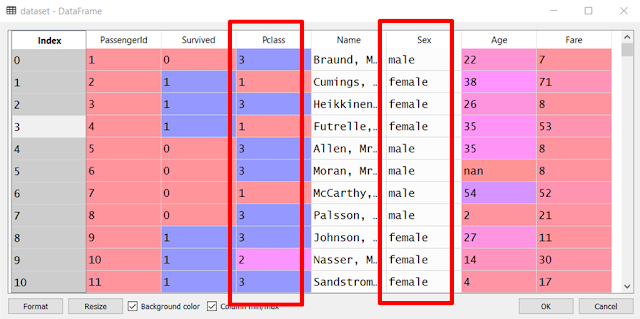# Separating independent and dependent variables

X = dataset.iloc[:, [2, 4]].values

y = dataset.iloc[:, 1].values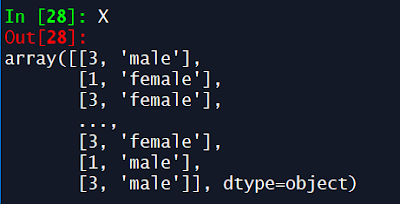# Encoding categorical data from sklearn.preprocessing import LabelEncoder, OneHotEncoder

labelencoder_X = LabelEncoder()

X[:, 1] = labelencoder_X.fit_transform(X[:, 1])

onehotencoder = OneHotEncoder(categorical_features = )

X = onehotencoder.fit_transform(X).toarray()

We have encoded the variable "Sex" in X which had two categorical values i.e. male and female. Hence, we've got 2 different columns. Third column in the picture below is for the variable "Pclass".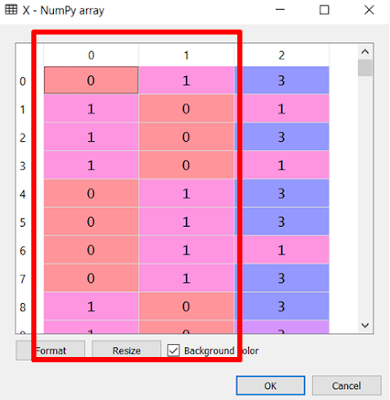# Splitting the dataset into the Training set and Test set
#from sklearn.cross_validation import train_test_split

X_train, X_test, y_train, y_test = train_test_split(X, y, test_size = 0.25, random_state = 0)# Feature Scaling

from sklearn.preprocessing import StandardScaler

sc = StandardScaler()

X_train = sc.fit_transform(X_train)

X_test = sc.transform(X_test
)

We did feature scaling as we want to obtain an accurate prediction of whether a passenger survived the sinking of titanic or not.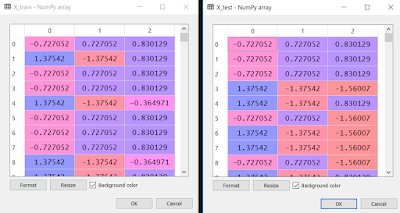# Fitting Logistic Regression to the Training set

from sklearn.linear_model import LogisticRegression

classifier = LogisticRegression(random_state = 0)

classifier.fit(X_train, y_train)

# Predicting the Test set results

y_pred = classifier.predict(X_test)# Making the Confusion Matrix

from sklearn.metrics import confusion_matrix

cm = confusion_matrix(y_test, y_pred)

Confusion Matrix helps to know how good our model is predicting. In other words, we will assess how correctly our Logistic Regression Model has learned the correlations from the training set to make accurate predictions on the test set.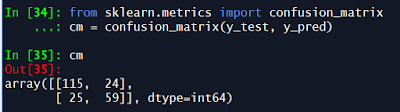Here, the diagonal with 115 and 59 shows the correct predictions and the diagonal 24 and 25 shows the incorrect predictions.

So, 115 + 59 = 174 are the total number of correct predictions out of 223 instances (in y_test)

Hence, our model showed 78% accuracy.